Concepts

Class 9
Chapter 8 Class 9 - Motion

Graphical Derivation of all 3 Equations of Motion

Our 3 equations of motion are

v = u + at

s = ut + 1 / 2at 2

v 2 - u 2 = 2as

Let's suppose an object with initial velocity u to final velocity v in time t. Let's derive all 3 equations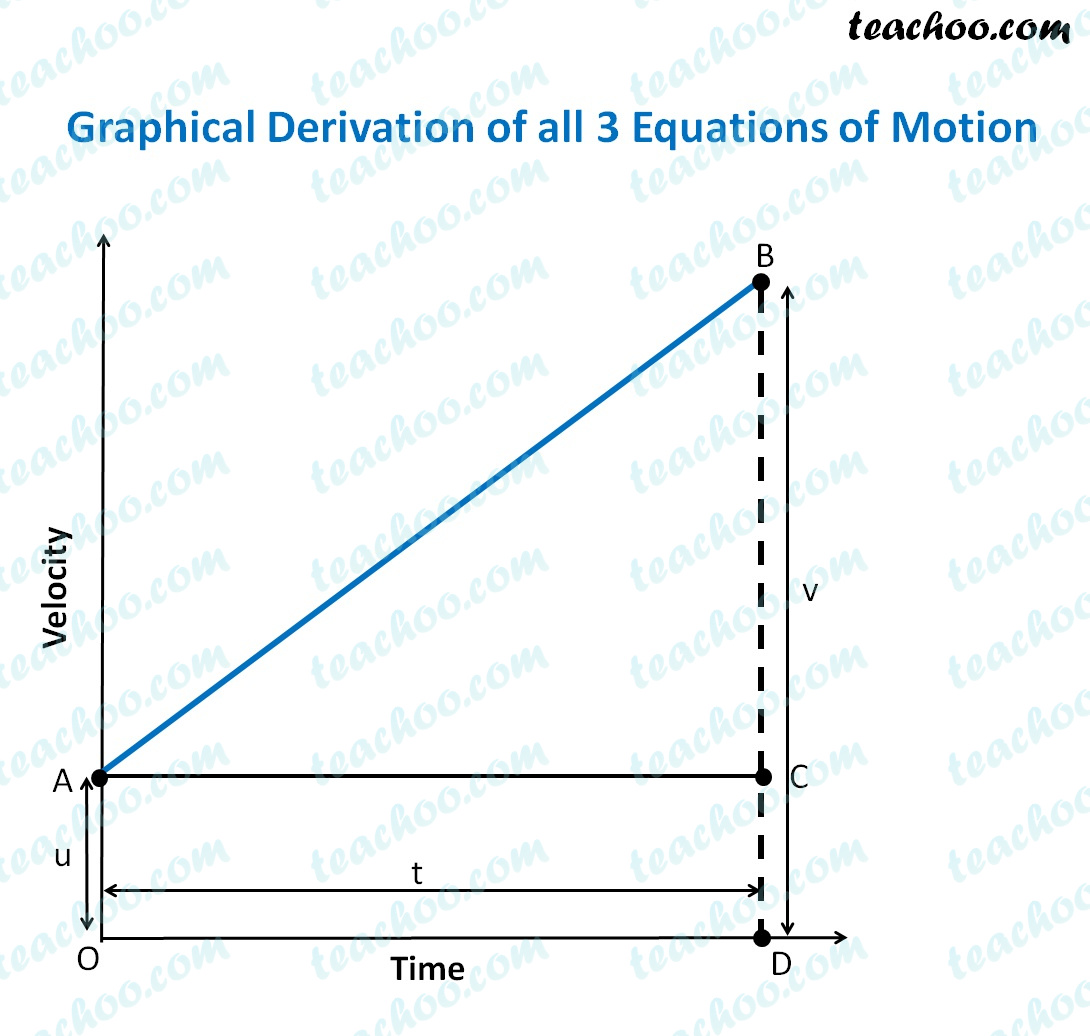Here,

Initial velocity = u = OA = CD

Final velocity = v = BD

Time taken = t = OD = AC

## First Equation of Motion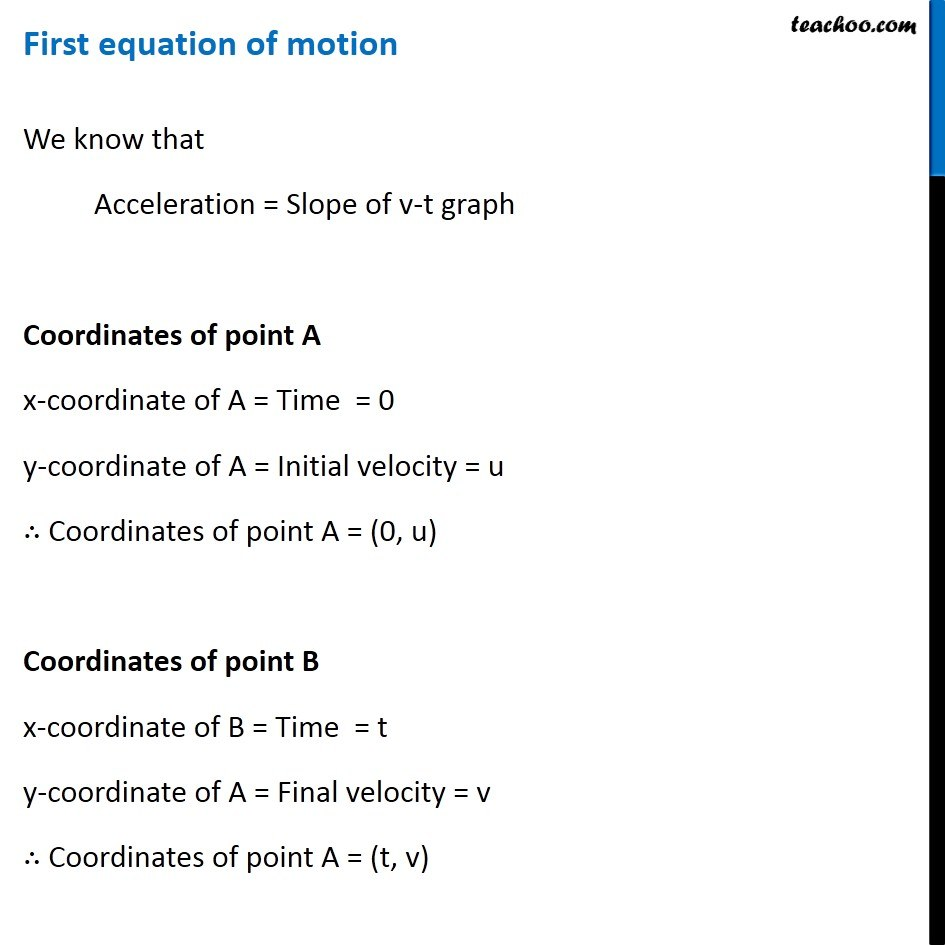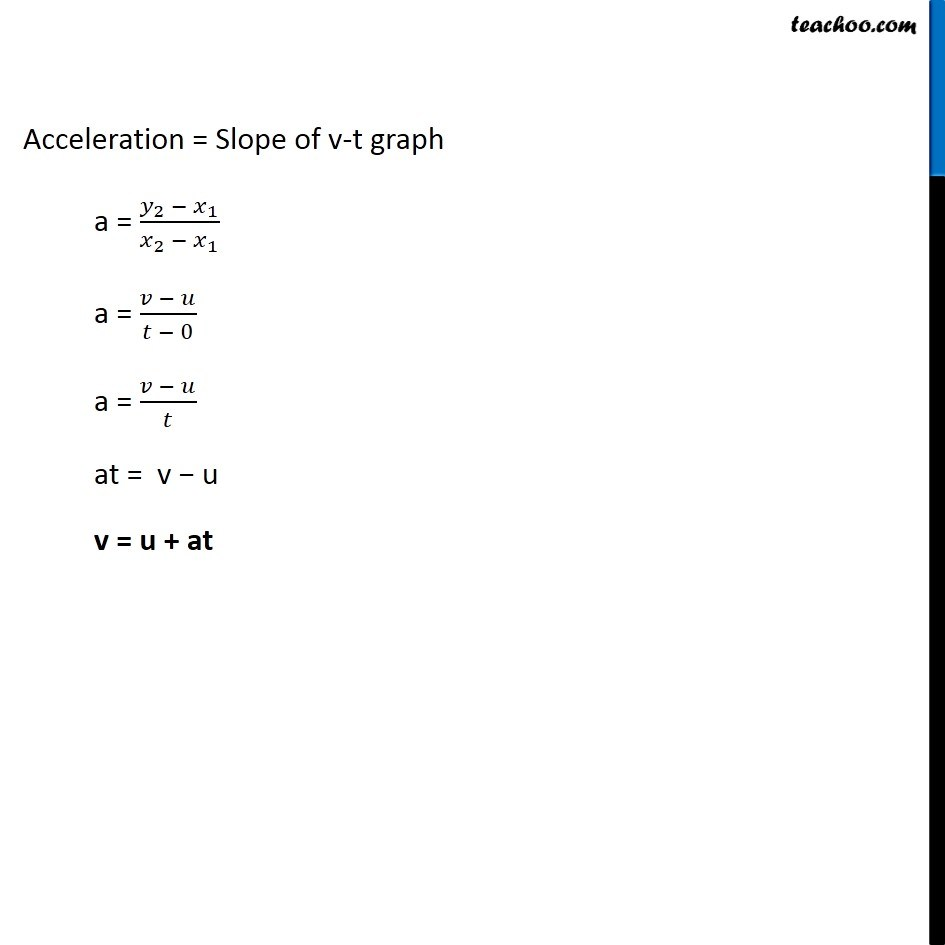## Second Equation of Motion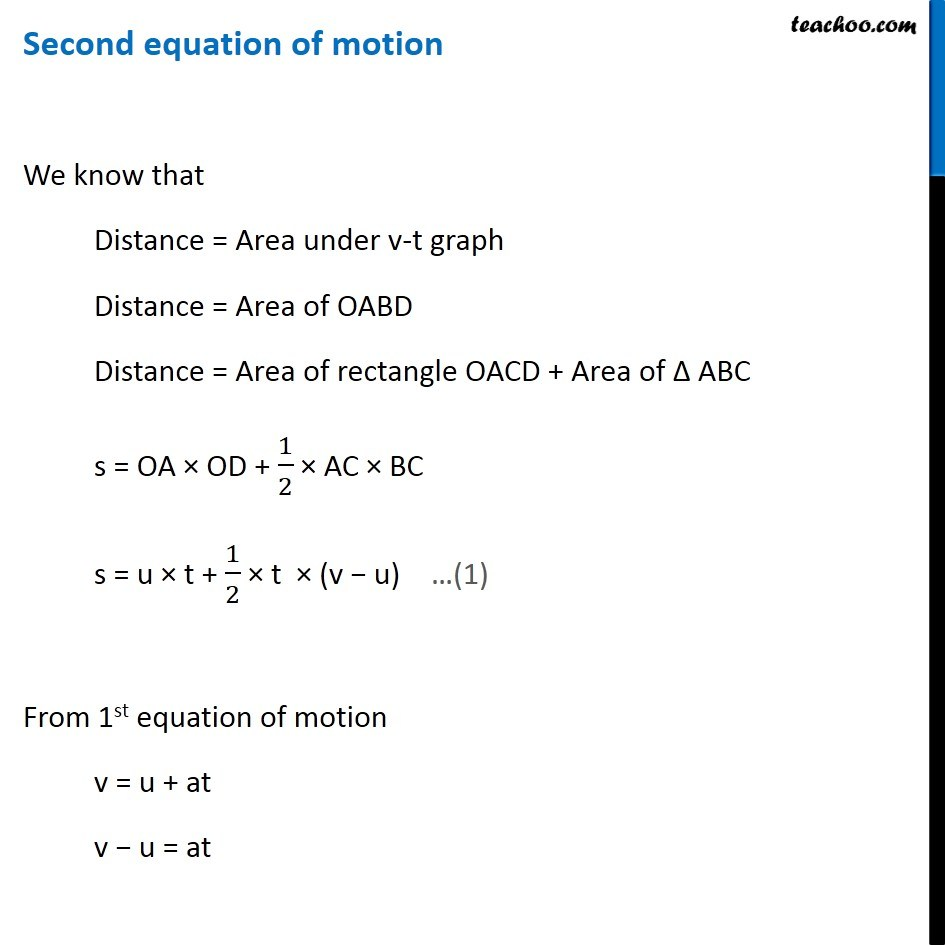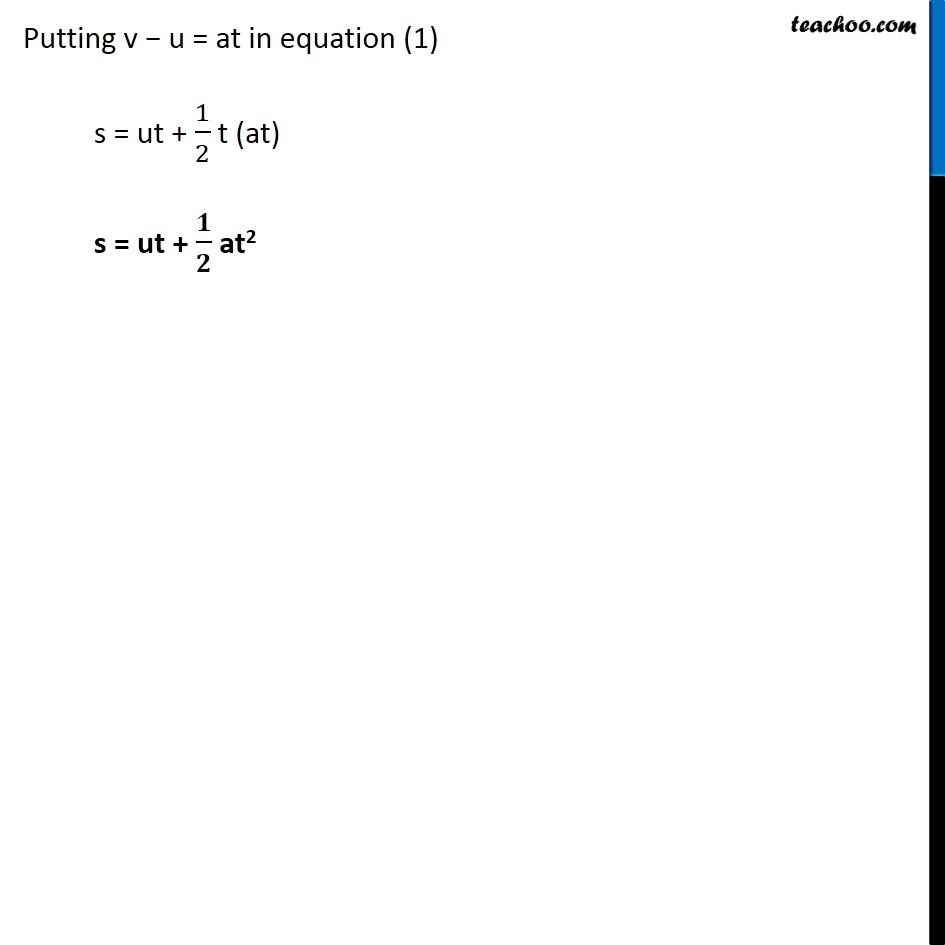## Third Equation of Motion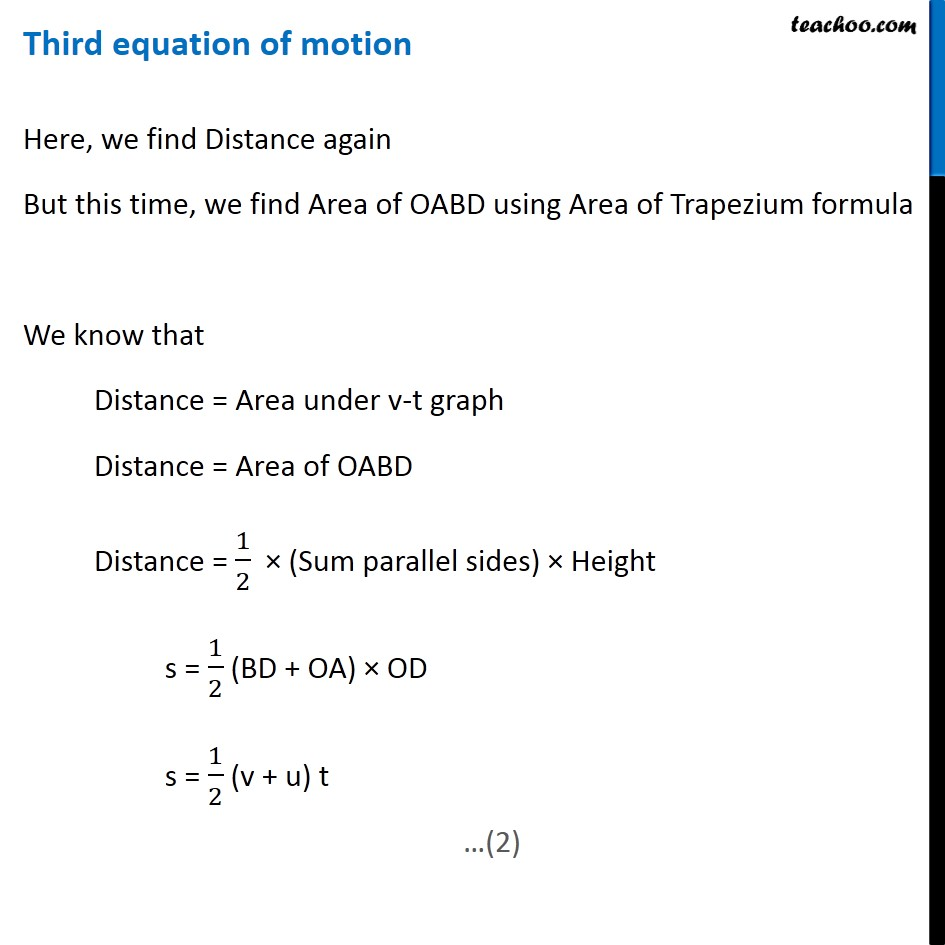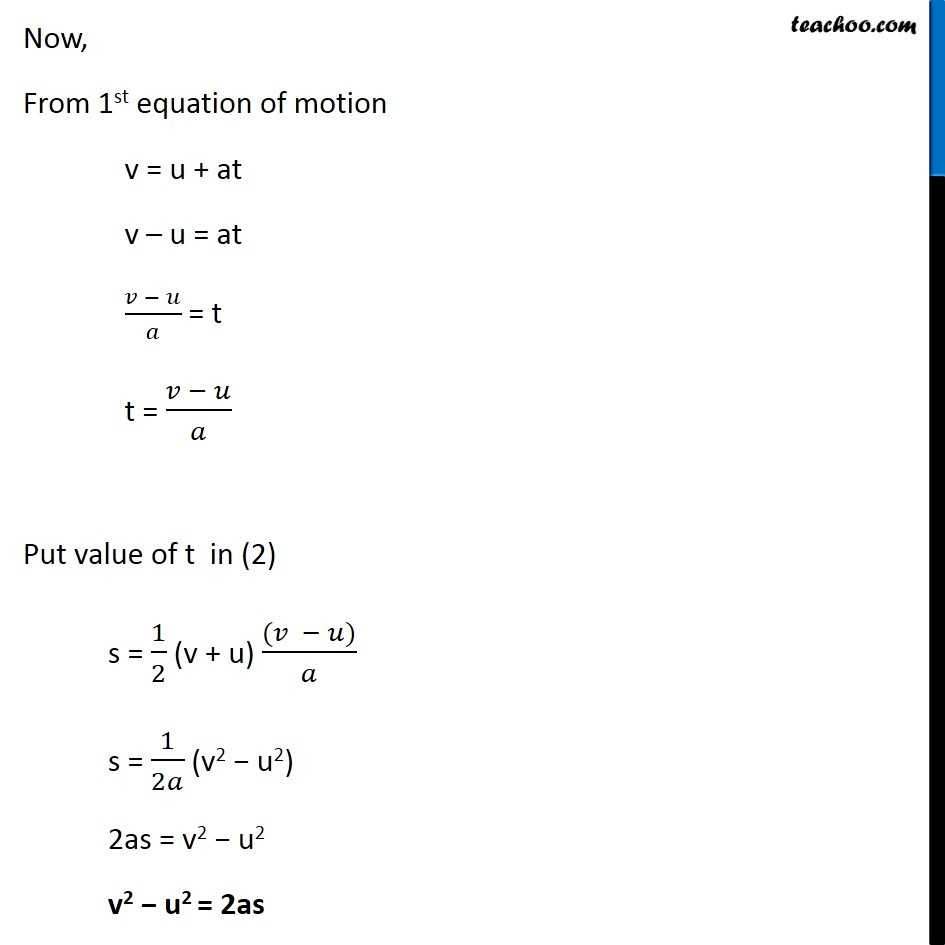Learn in your speed, with individual attention - Teachoo Maths 1-on-1 Class

### Transcript

First equation of motionWe know that Acceleration = Slope of v-t graph Coordinates of point A x-coordinate of A = Time = 0 y-coordinate of A = Initial velocity = u ∴ Coordinates of point A = (0, u) Coordinates of point B x-coordinate of B = Time = t y-coordinate of A = Final velocity = v ∴ Coordinates of point A = (t, v) Acceleration = Slope of v-t graph a = (𝑦_2 − 𝑥_1)/(𝑥_2 − 𝑥_1 ) a = (𝑣 − 𝑢)/(𝑡 − 0) a = (𝑣 − 𝑢)/𝑡 at = v − u v = u + at Acceleration = Slope of v-t graph a = (𝑦_2 − 𝑥_1)/(𝑥_2 − 𝑥_1 ) a = (𝑣 − 𝑢)/(𝑡 − 0) a = (𝑣 − 𝑢)/𝑡 at = v − u v = u + at Second equation of motion We know that Distance = Area under v-t graph Distance = Area of OABD Distance = Area of rectangle OACD + Area of Δ ABC s = OA × OD + 1/2 × AC × BC s = u × t + 1/2 × t × (v − u) From 1st equation of motion v = u + at v − u = at Putting v − u = at in equation (1) s = ut + 1/2 t (at) s = ut + 𝟏/𝟐 at2 Third equation of motion Here, we find Distance again But this time, we find Distance of OABD using Area of Trapezium formula We know that Distance = Area under v-t graph Distance = Area of OABD Distance = 1/2 × (Sum parallel sides) × Height s = 1/2 (BD + OA) × OD s = 1/2 (v + u) t Now, From 1st equation of motion v = u + at v – u = at (𝑣 − 𝑢)/𝑎 = t t = (𝑣 − 𝑢)/𝑎 Put value of t in (2) s = 1/2 (v + u) ((𝑣 − 𝑢))/𝑎 s = 1/2𝑎 (v2 − u2) 2as = v2 − u2 v2 − u2 = 2as